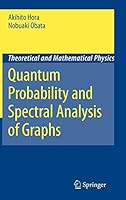#### Quantum Probability and Spectral Analysis of Graphs (Theoretical and Mathematical Physics)

Quantum Probability and Spectral Analysis of Graphs (Theoretical and Mathematical Physics)